Function Repository Resource:

# TapeDiagram

Visualize fractions, integers and multiplication using tape diagrams

Contributed by: Wolfram|Alpha Math Team
 ResourceFunction["TapeDiagram"][n/d] creates a tape diagram representing a single fraction n/d. ResourceFunction["TapeDiagram"][m] creates a tape diagram representing a single whole number m. ResourceFunction["TapeDiagram"][n/d*m] creates a tape diagram representing the multiplication of a whole number m by a fraction n/d. ResourceFunction["TapeDiagram"][n*m] creates a tape diagram representing the multiplication of two whole numbers m and n. ResourceFunction["TapeDiagram"][{expr1,expr2,…}] creates a stack of tape diagrams.

## Details

ResourceFunction["TapeDiagram"] accepts inputs greater than 1/200 and less than 200.
ResourceFunction["TapeDiagram"] has the same options as Graphics, with the following additions:
 "BracketPosition" Automatic controls the placement of the brackets and their labels "DotAfterIntegerPart" Automatic shows a dotted outline around the fractional part of the represented number FontColor Black sets the font color of the text in the tape diagram FillingStyle RGBColor[0.99,0.9,0.5] sets the color for the filled part of the tape diagram "IntegerMarkers" False draws thick lines at intervals of one throughout the tape "TapeColor" RGBColor[0.95,0.95,0.95] sets the color for the unfilled part of the tape diagram "SubdivisionWidth" None adds dashed lines at intervals of a given width "UnitLabels" Automatic sets the text of the label displayed in the sections of the tape diagram
The following settings for the "BracketPosition" option can be given:
 Top places the brackets and labels on top of the tape diagram Bottom places the brackets and labels on the bottom of the tape diagram None removes brackets and their labels Automatic for tape diagrams showing multiplication of a fraction by a whole number, one bracket appears on top and the other on the bottom
"SubdivisionWidth" can be set to a positive numeric value.
ResourceFunction["TapeDiagram"] has the attribute HoldAll.

## Examples

### Basic Examples (3)

Create a tape diagram representing a single fraction:

 In:=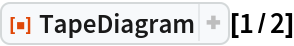Out=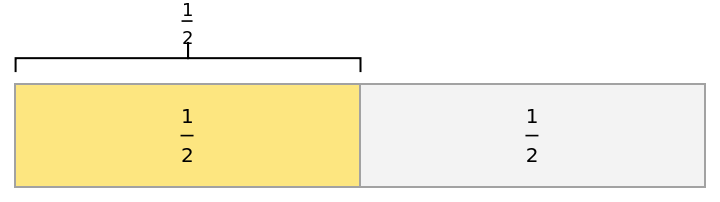Create a tape diagram representing the multiplication of a fraction and a whole number:

 In:=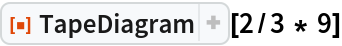Out=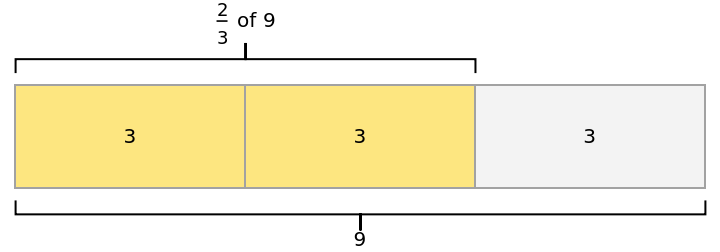Create a stack of tape diagrams:

 In:=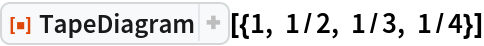Out=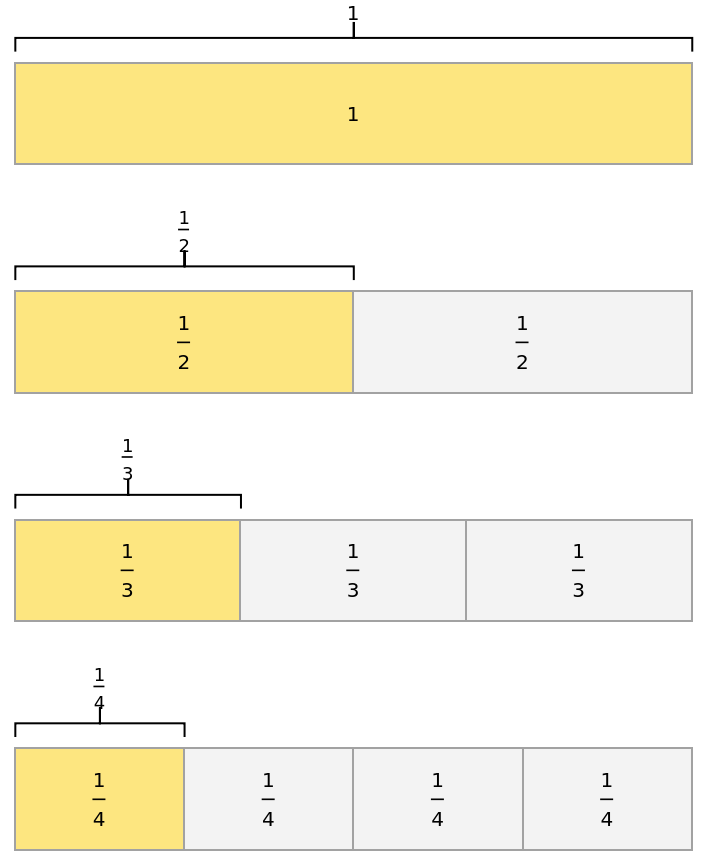### Scope (2)

Create a tape diagram representing an improper fraction:

 In:=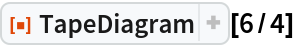Out=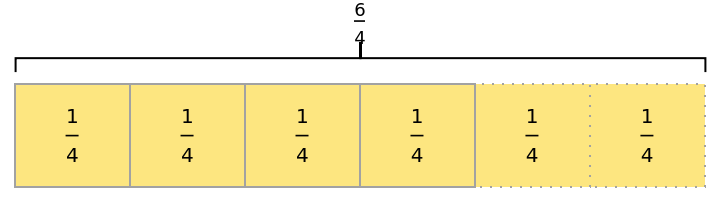Create a tape diagram representing the multiplication of two whole numbers:

 In:=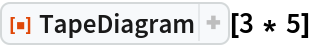Out=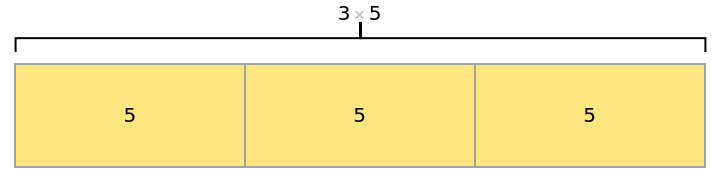### Options (18)

#### BracketPosition (3)

Move the brackets to the Top or Bottom of the tape:

 In:=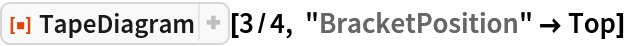Out=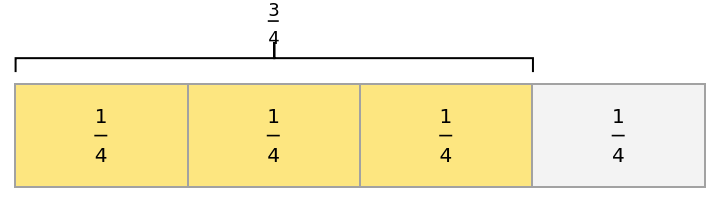In:=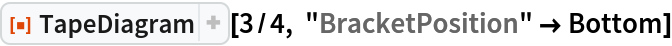Out=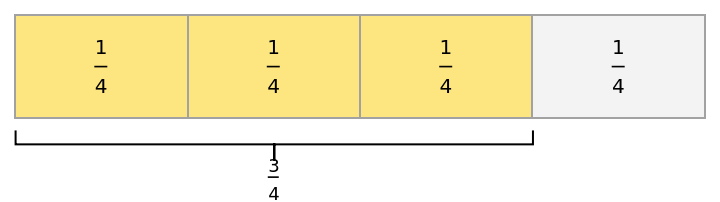Use None to show a tape with no brackets:

 In:=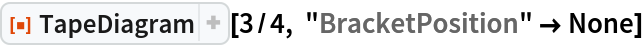Out=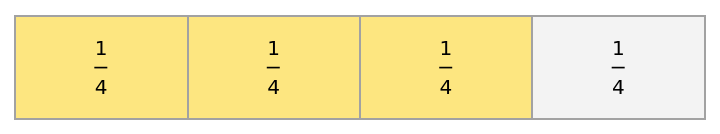For tapes showing multiplication of a fraction by a whole number, both brackets are adjusted:

 In:=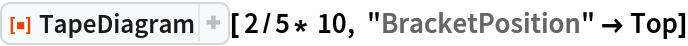Out=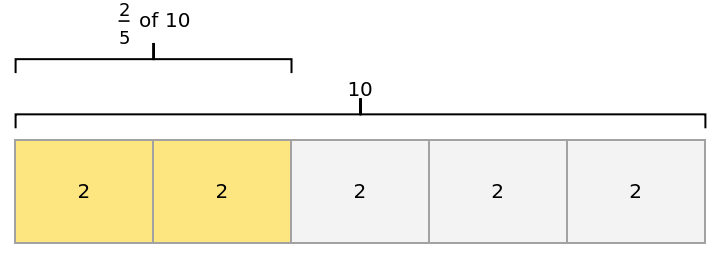In:=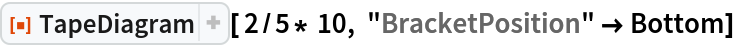Out=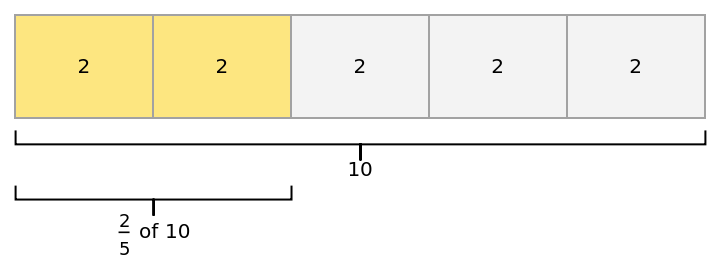#### DotAfterIntegerPart (4)

For a single fraction with sufficiently wide divisions, the tape automatically becomes dotted after the largest full integer:

 In:=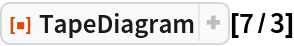Out=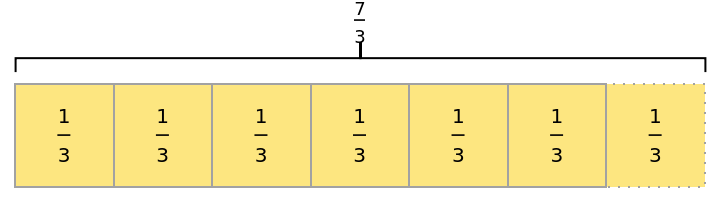When the tape divisions are small, the dotting is automatically turned off:

 In:=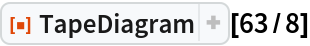Out=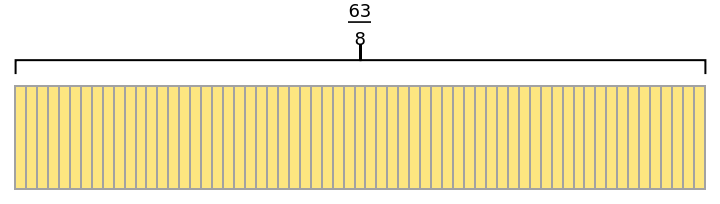"DotAfterIntegerPart" can be set to True or False to control when the dotting appears:

 In:=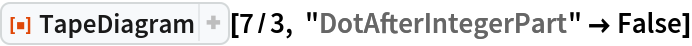Out=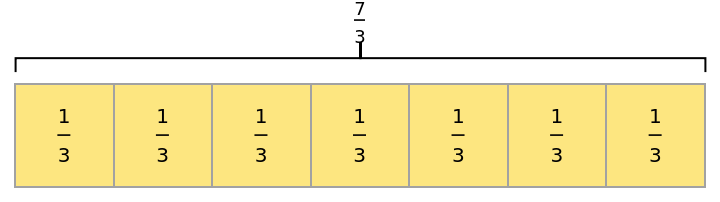In:=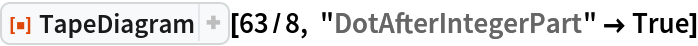Out=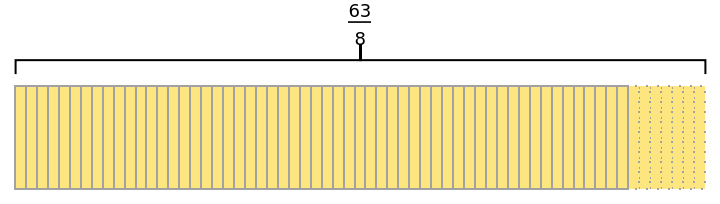For tapes representing multiplication of a fraction by a whole number, the dotting begins after a length representing the given whole number:

 In:=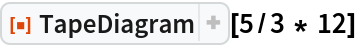Out=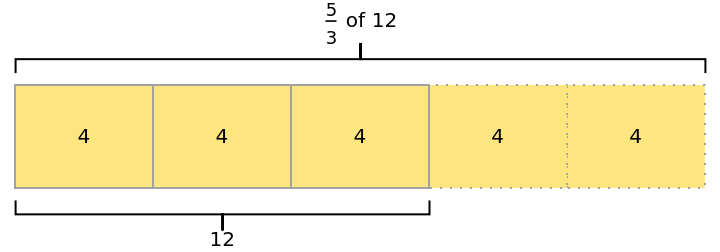#### IntegerMarkers (1)

Emphasize intervals of length one by setting "IntegerMarkers" to True:

 In:=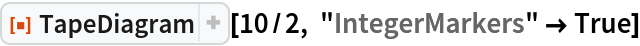Out=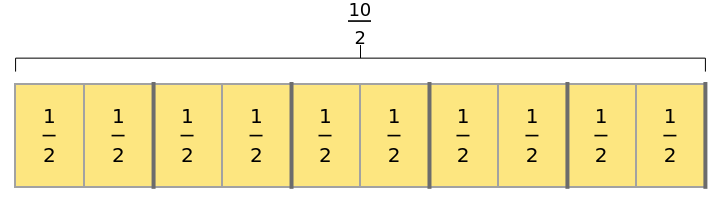In:=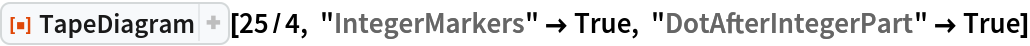Out=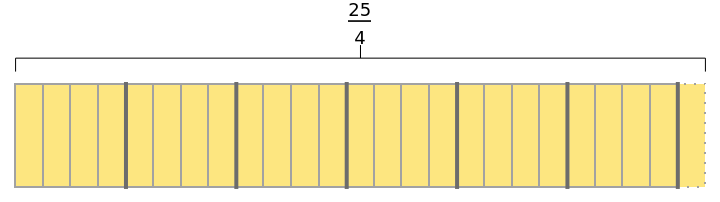#### FillingStyle (1)

Change the filling style of the tape:

 In:=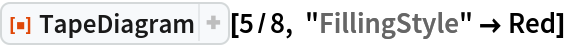Out=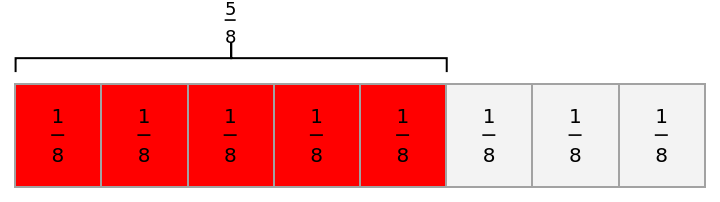#### TapeColor (1)

Change the color of the tape itself:

 In:=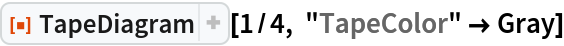Out=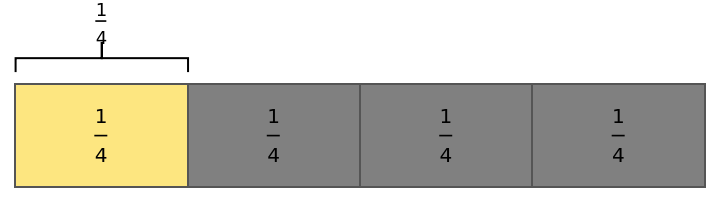In:=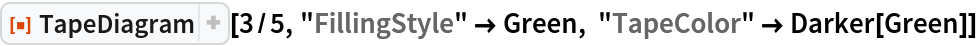Out=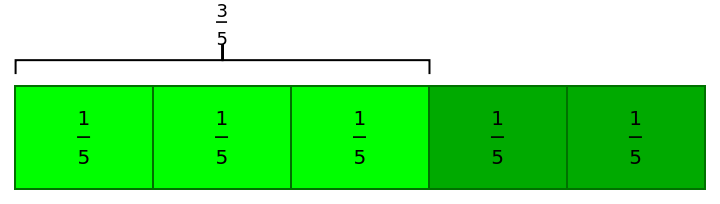#### FontColor (1)

Change the font color of the tape labels:

 In:=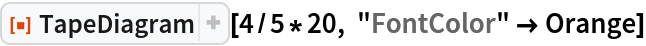Out=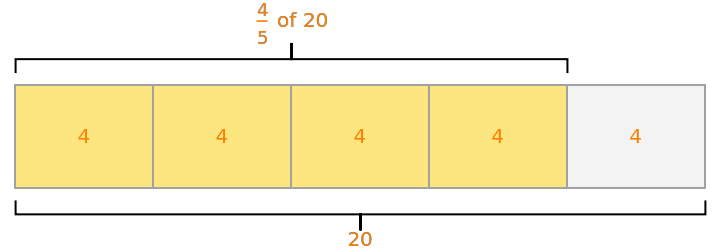#### SubdivisionWidth (2)

The "SubdivisionWidth" option allows the user to add additional subdivisions to the tape, for example, visualizing subdivisions of width 1/8 on a tape representing 1/2:

 In:=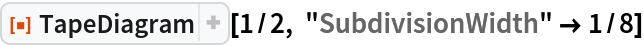Out=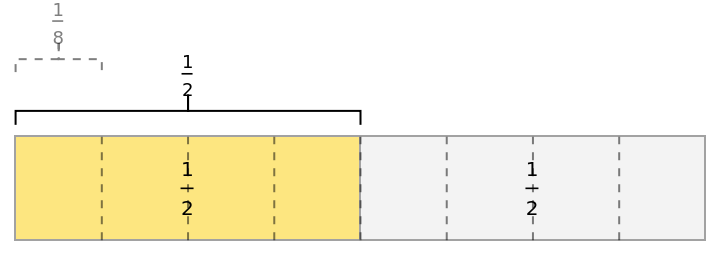Setting a "SubdivisionWidth" for a stack of tape diagrams applies the subdivision to all tapes at once:

 In:=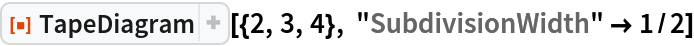Out=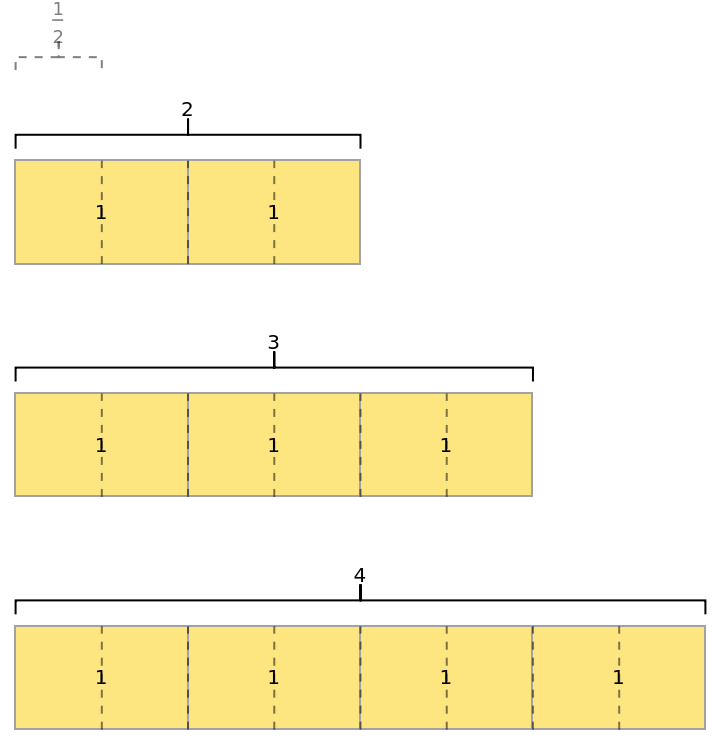#### UnitLabels (5)

Setting the "UnitLabels" option to False removes the labels that show in each section of the tape:

 In:=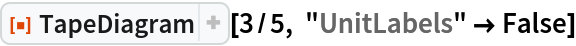Out=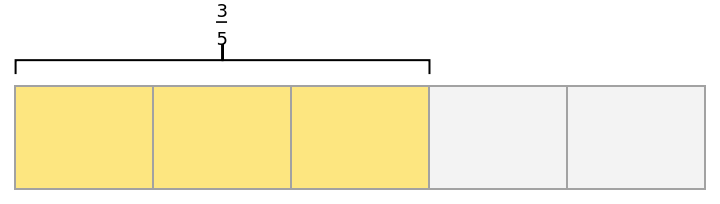Setting the "UnitLabels" option to Tooltip removes the labels that show in each section of the tape and also adds a Tooltip showing the value of each section:

 In:=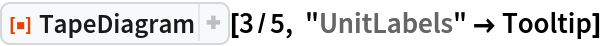Out=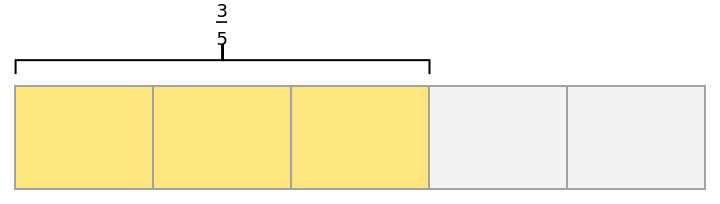For tapes that are small, the "UnitLabels" option is automatically set to Tooltip:

 In:=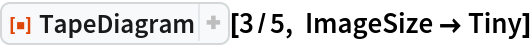Out=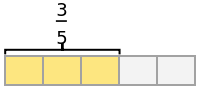Define custom unit labels by setting the value of "UnitLabels" to any string:

 In:=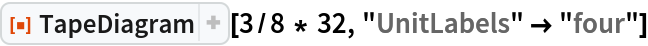Out=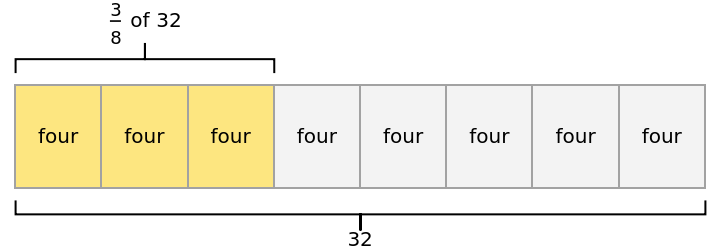In:=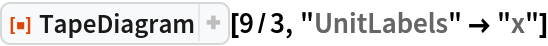Out=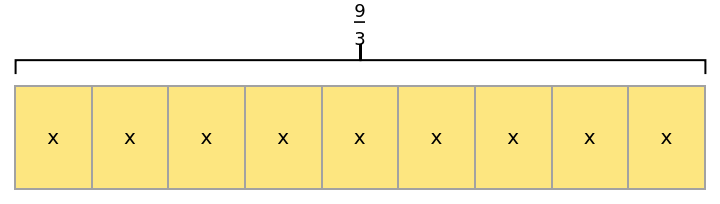For stacks of tapes, "UnitLabels" can be set individually for each tape by providing a list of strings or a list of functions:

 In:=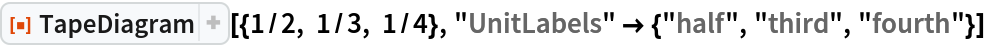Out=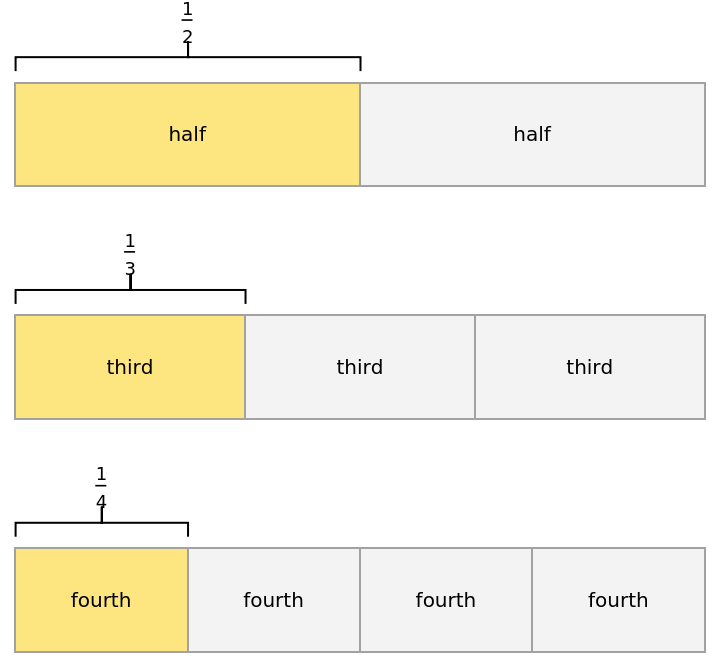## Publisher

Wolfram|Alpha Math Team

## Version History

• 2.0.0 – 23 March 2023
• 1.0.2 – 28 March 2022
• 1.0.1 – 23 March 2022
• 1.0.0 – 08 June 2021

## Author Notes

To view the full source code for TapeDiagram, evaluate the following:

 In:=